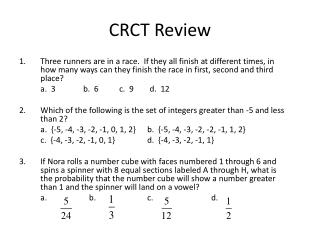DownloadDownload PresentationCRCT Review

# CRCT Review

Download Presentation## CRCT Review

- - - - - - - - - - - - - - - - - - - - - - - - - - - E N D - - - - - - - - - - - - - - - - - - - - - - - - - - -
##### Presentation Transcript

1. CRCT Review • Three runners are in a race. If they all finish at different times, in how many ways can they finish the race in first, second and third place? a. 3 b. 6 c. 9 d. 12 • Which of the following is the set of integers greater than -5 and less than 2? a. {-5, -4, -3, -2, -1, 0, 1, 2} b. {-5, -4, -3, -2, -2, -1, 1, 2} c. {-4, -3, -2, -1, 0, 1} d. {-4, -3, -2, -1, 1} • If Nora rolls a number cube with faces numbered 1 through 6 and spins a spinner with 8 equal sections labeled A through H, what is the probability that the number cube will show a number greater than 1 and the spinner will land on a vowel? a. b. c. d.

2. Parallel Lines • Two lines with the same slope are said to be parallel lines. If you graph them they will never intersect. • We can decide algebraically if two lines are parallel by finding the slope of each line and seeing if the slopes are equal to each other. • We can find the equation of a line parallel to a given line and going through a given point by: • a) first finding the slope m of the given line; • b.) finding the equation of the line through the given point with slope m.

3. Testing if Lines are Parallel Are the lines parallel? Find the slope of The slope m = -4 The slope m = -4 Find the slope of Since the slopes are equal the lines are parallel.

4. Practice Testing if Lines are Parallel Are the lines parallel? Since the slopes are different the lines are not parallel. parallel? Are the lines Since the slopes are equal the lines are parallel.

5. Parallel Equations • Parallel lines have the same slope • Given: y = 3x + 6, write an equation parallel that passes through (4, 7) m = 3. Use y = mx + b to find the other equation. Substitute m =3; x = 4; y = 7 to find b. 7 = 3(4) + b 7 = 12 + b -12 -12 -5 = b The new equation with point (4,7) is: y = 3x - 5

6. Testing if Lines Are Perpendicular Since the slopes are negative reciprocals of each other the lines are perpendicular.

7. Practice Testing if Lines Are Perpendicular Since the slopes are not negative reciprocals of each other (their product is not -1) the lines are not perpendicular Since the slopes are negative reciprocals of each other (their product is -1) the lines are perpendicular.

8. Perpendicular Equations • Perpendicular lines have the “opposite reciprocal” slope. • Given: y = -3x + 1; write an equation perpendicular passing through (6, -1) So, m = 1/3. Use y = mx + b to find the other equation. Substitute m = 1/3; x = 6; y = -1 to find b. -1 = 1/3(6) + b -1 = 6/3 + b -1 = 2 + b -2 -2 -3= b The new equation with point (6,-1) is: y = 1/3x - 3